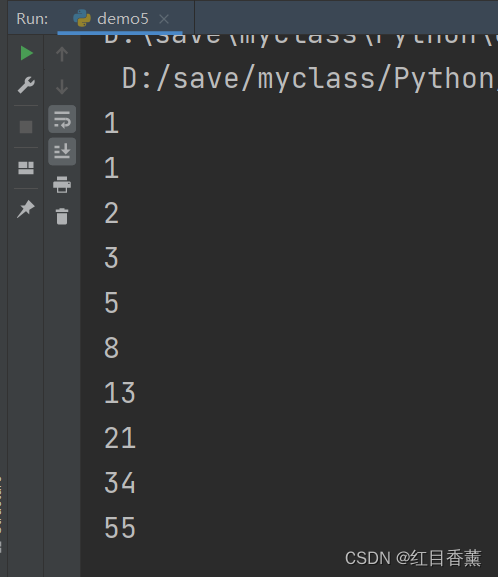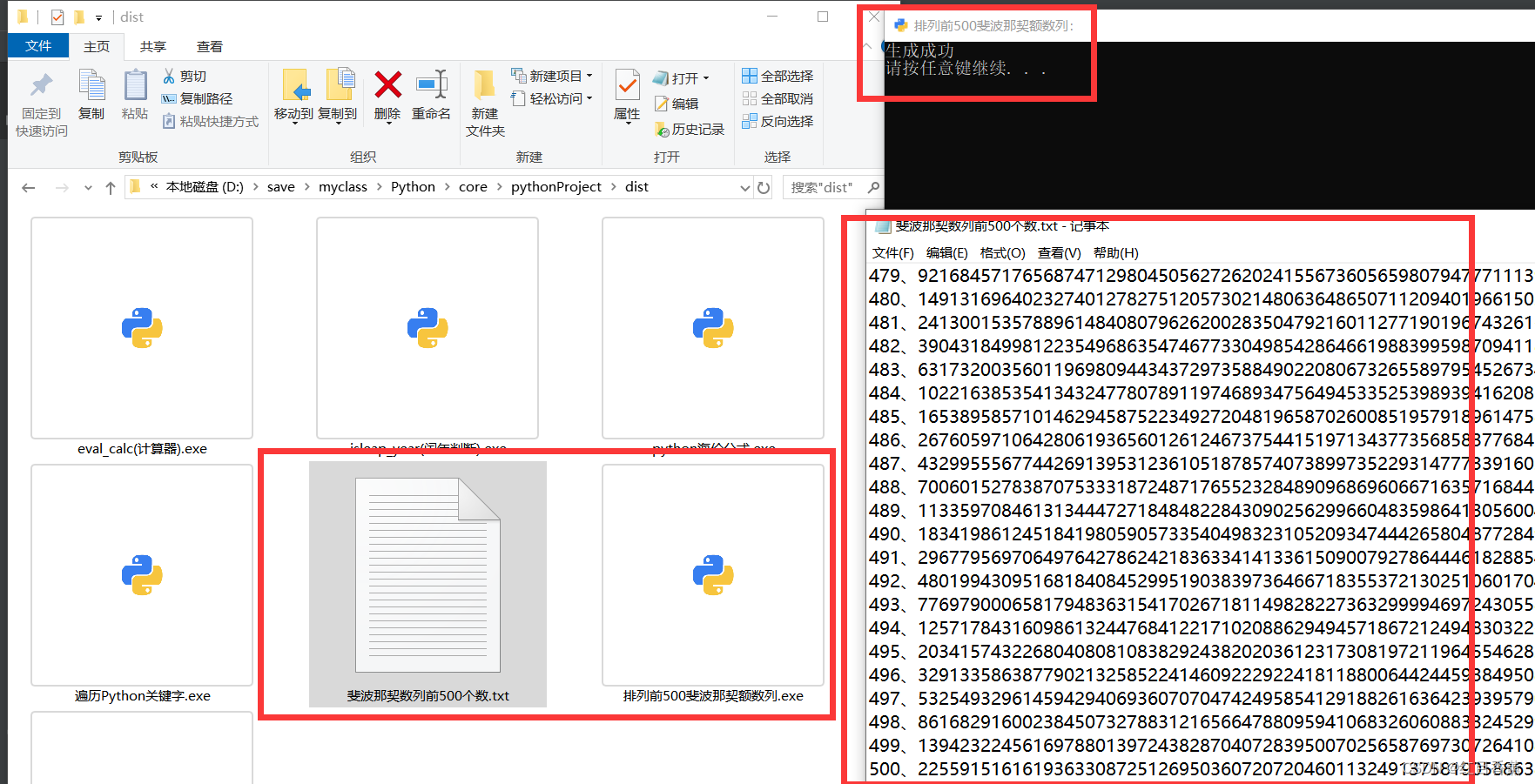> 文档中心 > Python数学计算工具3、Python 斐波那契数列-前500项列表

# Python数学计算工具3、Python 斐波那契数列-前500项列表

``one = 0two = 1nth = 1for i in range(0, 10):    print(nth)    nth = one + two    one = two    two = nth````def dfs(n):    if n == 1 or n == 2: return 1    return dfs(n - 1) + dfs(n - 2)print(dfs(10))``

``# 排列前500斐波那契额数列import osos.system("title 排列前500斐波那契额数列：")one = 0two = 1nth = 1str_list = []for i in range(0, 500):    nth = one + two    one = two    two = nth    str_list.append(nth)count = 0str1 = []with open("斐波那契数列前500个数.txt", "w+", encoding="utf-8") as file:    for item in str_list: count += 1 str1.append("{0}、{1}\n".format(count, item))    file.writelines(str1)    file.flush()``

pyinstaller -F -p D:\save\Exe\studys\Python\exe\Lib -i D:\save\myclass\Python\core\pythonProject\python.ico demo5.py -n " 排列前500斐波那契额数列"